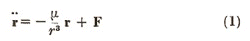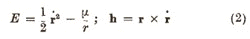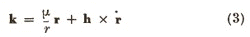# Satellite Orbit Perturbations in Vector Form

## Abstract

THE first-order perturbation theory of satellite orbits can be formulated simply and elegantly in terms of constants of the unperturbed motion using vector methods. For let the equation of motion be:where μ = GM (M = mass of the earth), and F is a general perturbing force (per unit mass). The energy E and angular momentum h (both per unit mass) defined as:are constants of the unperturbed motion. Hamilton's integral, which may be written in the form1:provides a further constant of the unperturbed motion under an inverse square law force. Taking scalar products with h and r, k is seen to be a vector of length μe lying along the major axis in the direction of apogee. The orbit is over-determined by E, h and k which give seven scalar equations.

## Access options

from\$8.99

All prices are NET prices.

## References

1. 1

Milne, E. A., Vectorial Mechanics, 236 (Interscience Pub., New York, 1948).

2. 2

King-Hele, D. G., Proc. Roy. Soc., A, 247, 49 (1958).

3. 3

Herrick, S., Pub. Astron. Soc. Pacific, 60, 321 (1948).

4. 4

Musen, P., J. Geophys. Res., 65, 1391 (1960).

## Rights and permissions

Reprints and Permissions

ALLAN, R. Satellite Orbit Perturbations in Vector Form. Nature 190, 615 (1961). https://doi.org/10.1038/190615a0

• Issue Date:

• ### Celestial mechanics with geometric algebra

• David Hestenes

Celestial Mechanics (1983)

• ### Radiation forces on small particles in the solar system

• Joseph A. Burns
• , Philippe L. Lamy
•  & Steven Soter

Icarus (1979)

• ### Studies in the application of recurrence relations to special perturbation methods

• A. E. Roy
•  & P. E. Moran

Celestial Mechanics (1973)

• ### The effect of aerodynamic lift on satellite orbits

• G.E. Cook

Planetary and Space Science (1964)

• ### On the secular variations of the elements of satellite orbits

Proceedings of the Royal Society of London. Series A. Mathematical and Physical Sciences (1962)